# Excel Formulas for Beginners: Bring Your Spreadsheet to Life

AUTHOR:  JASON MORRELLminutes remaining

Formulas for beginners is one of the hardest parts of Excel. At least in your head!

Formulas simply allow you to manipulate date in Excel. That could be something as simple as adding up a series of numbers or at the other extreme, formulas can be used for complicated forecasting or technical engineering equations.

In this post you'll discover how to get started and create some simple formulas of your own. Welcome to this post.

## 1. What you need to know about every formula (part 1)

• Every formula must start with a '=' (found next to the BACKSPACE key).
• The usual mathematical operators can be used

-    (subtract)
*    (multiply - don't use x)
/    (divide - there is no ÷ symbol on the keyboard!)
• Formulas can use a mixture of

■    Plain numbers, called Constants (e.g. 5)
■    Cell references (e.g. A3)
■    Cell ranges (e.g. A1:E5)

For example, =1+2+3+4 and =A1+A2+A3 are both valid formulas.

Be careful. The = is on the same key as the + and it’s easy to type something like =2=2 instead of =2+2. In this instance, the two numbers won’t be added, but rather they will be compared against each other to see if they are the same. If they match, the answer is TRUE. If they don’t match, the answer is FALSE.

## 2. What you need to know about formulas (part 2)

The steps of a formula are always calculated in a set order. These are called the Order of Operations and they were established in the 17th century to ensure everybody sings from the same sheet and any ambiguity is eliminated.

Here's an example.

Many people think the answer to =2+3*4 is 20, when in fact the correct answer is 14.

Let me explain.

The BEDMAS acronym shows the order in which parts of a formula are calculated. Excel uses symbols to represent mathematical operators. The table below explains what BEDMAS stands for and shows what symbols Excel uses.

• B = Brackets      ( )
• E = Exponentiation (to the power of)     ^
• D = Division     /
• M = Multiplication     *
• A = Addition     +
• S = Subtraction     -

The equation 2 + 2 * 2 + 2 gives an answer of 8, not 10.

The equation 100 – 50 * 2 gives an answer of zero, not 100.

The equation 2 + 3 * 4 gives an answer of 14, not 20.

Let's break this last one down.

Following the BEDMAS sequence, the multiplication segment (3 * 4) is calculated before the addition segment. So, 3 * 4 = 12, then 2 is added to give an answer of 14.

We could change the formula to give an answer of 20 by adding brackets.

(2 + 3) * 4 equals 20, because the part inside the - brackets is done first.

## 3. How to enter a formula in Excel

### Method 1: Type directly into a cell

Single-click a blank cell, then type a formula. This overwrites the existing contents of the cell.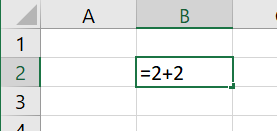OR

### Method 2: Use the formula bar

The formula bar is the large white box located between the toolbars and the column headers and to the right of the  button.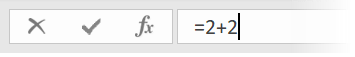Select a cell, click in the formula bar and type a formula.

When you are entering or modifying a formula, a cross (X) and a tick (✓) appear next to the Fx button on the formula bar.

• Click the ✓ or press Enter to confirm the formula.
• Click the X or press Esc to cancel any changes you have made.

## 4. How to edit a formula

Any of the following techniques can be used to edit the contents of a cell.

• Double click a cell to edit the formula within the cell.
• Single-click a cell and press F2 to edit the formula within the celł.
• Single-click a cell then click in the formula bar to edit the formula there.

## 5. How to use Smart Tags

When there is an error, a small coloured triangle is placed in the corner of the cell.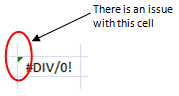When the cell is selected, a Smart tag appears.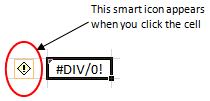When the Smart tag is clicked, options are provided to help resolve the error.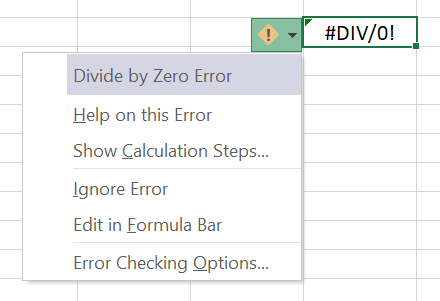## 6. Common errors in beginners formulas

### #DIV/0! Error

Formula is attempting to divide by zero. Check that cell references are not empty and do not contain zero.

### #NUM! Error

The result of the formula is invalid (e.g. =1000^1000 cannot be represented as the answer is too large.

### #REF! Error

Formula is attempting to reference a cell that is no longer valid. Check that the referenced cell has not been deleted or moved.

NB. Not to alarm you, but these are just three of the most common errors. There are many other errors which you will probably come across, given enough time!

## 7. Key Takeaways

• To create a new formula, select a cell and start typing.
• To accept a formula, press ✓ or Enter.
• To cancel a formula, press X or Esc (Escape).
• To edit a formula, double-click cell or select a cell and press F2 or click directly in the formula bar.
• Every formula must start with '='.
• Every formula will work according to the BEDMAS sequence.
• To find the cause of an error click the cell with the error then click the smart tag next to it.

Please feel free to post any questions you have into the comments below. And before you leave this page, I want to give you one quick little action item!

ACTION ITEM: Comment below with ONE thing you picked up from this post and share how it has helped, or will help, you. It's good to share your successes because it encourages others and provides an instant boost.

Enjoy the rest of your day!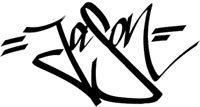Jason Morrell

Jason loves to simplify the hard stuff, cut the fluff and share what actually works. Things that make a difference. Things that slash hours from your daily work tasks. He runs a software training business in Queensland, Australia, lives on the Gold Coast with his wife and 4 kids and often talks about himself in the third person!

SHARE

POPULAR EXCEL POSTS

Excel screen: A beginners tour

How many row and columns does Excel have?

Formulas quick start guide

3 ways to calculate an average in Excel

How to calculate percentages

3 places to find help in Excel

14 easy ways to make your spreadsheet look fantastic

Smart ways to resize multiple columns at once

Excel's COUNT functions

«« SEE ALL EXCEL POSTS »»Master Microsoft Office
in 30 days or less

more from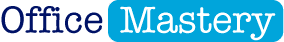## How to fix formulas: 21 Fatal Formula Fails (and how to avoid them)

How to fix formulas: 21 Fatal Formula Fails (and how to avoid them)

## How to master the VLOOKUP formula in Excel

How to master the VLOOKUP formula in Excel

## Budgeting 101: Your Easy Guide to Creating a Business Budget in Excel

Budgeting 101: Your Easy Guide to Creating a Business Budget in Excel

## How many rows and columns does Excel have?

How many rows and columns does Excel have?

JOIN THE DISCUSSION

{"email":"Email address invalid","url":"Website address invalid","required":"Required field missing"}